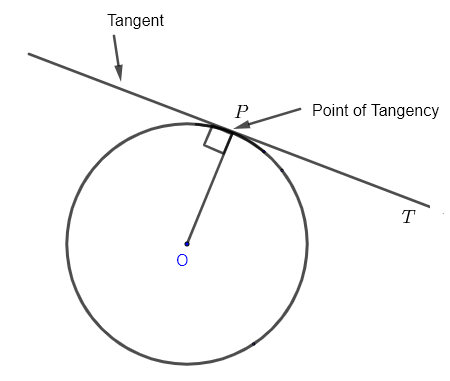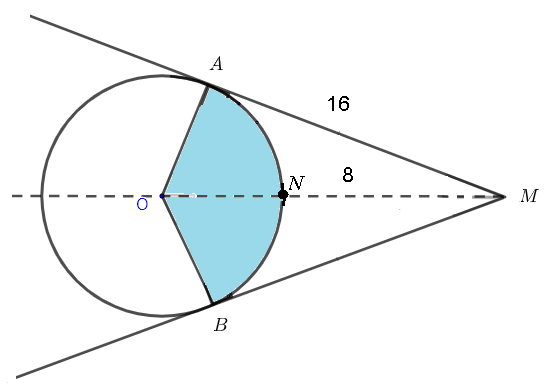# Tangents to a Circle with Questions and Solutions

## Tangent to a Circle

A tangent to circle touches the circle at one point only. In the figure below the tangent $T$ cuts the circle at point $P$ called the point of tangency.An important property of the tangent to a circle it that the tangent $T$ and the radius $OP$ are perpendicular.

## Two Intersecting Tangents to a Circle

In the figure below, $MA$ and $MB$ are tangent to the same circle with center $O$. The two tangents intersect at point $M$The most important properties are

1. $MO$ is an angle bisector to $\angle AMB$
2. $OM$ is an angle bisector to $\angle AOB$
3. the right triangles $\triangle AMO$ and $\triangle BMO$ are congruent.

## Questions With Solutions

Question 1
In the figure below, $MA$ and $MB$ are tangent to the circle with center $O$. $MO$ cuts the circle at point $N$ such that the length of $MN$ is equal to $8$ units and the length of $MA$ is equal to $16$
1) Find the radius of the circle.
2) Find the size of angle $AOB$.
3) Find the area of the shaded (in blue) sector.Solution
1) The tangent $MA$ makes an angle of $90^{\circ}$ with the radius $OA$ and therefore $OAM$ is a right triangle. Using the Pythagorean theorem, we write the equation: $\quad OA^2 + AM^2 = (8 + ON)^2$
Let $r = OA = ON$ be the radius of the circle and rewrite the above equation as: $\quad r^2 + 16^2 = (8 + r)^2$
Expand the right side of the equation: $\quad r^2 + 16^2 = 8^2 + 16 r + r^2$
Group like terms, simplify and rewrite the above equation as: $\quad 16^2 - 8^2 = 16 r$
Solve the above for $r$ to obtain: $\quad r = 12$
2) Let us first find the size of angle $AOM$ using the tangent formula: $\quad \tan \angle AOM = \dfrac{AM }{OA} = \dfrac{16}{12} = \dfrac{4}{3}$
Hence: $\quad \angle AOM = \arctan (4/3)$
$OM$ bisects $\angle AOB$, hence $\quad \angle AOB = 2 \angle AOM = 2 \arctan(4/3)$
3) Use the formula of the area of a sector to calculate the area $A_s$ of the shaded sector as: $\quad A_s = (1/2) \times \angle AOM \times r^2 = (1/2) \times 2 \arctan(4/3) \times 12^2 \approx 133.53$ square units

Question 2
In the figure below, $MA$ and $MB$ are tangent to the circle with center $O$. $MO$ cuts the circle at point $N$ and the length of the arc $ANB$ is equal to $22$ units. The radius of the circle is equal to $8$.
Find the distance from $N$ to $M$.Solution
Let $r$ be the radius of the circle. The length of the arc $ANB$ is given by the formula: $S = \angle AOB \times r$
Solve the above for $\angle AOB$: $\quad \angle AOB = \dfrac{S}{r} = \dfrac{22}{8}$
$OM$ bisects angle $AOB$; hence $\quad \angle AOM = \dfrac{\angle AOB}{2} = \dfrac{22}{16}$
Use the right triangle $OAM$ to write: $\cos \angle AOM = \dfrac{r}{OM}$
Hence: $OM = \dfrac{r}{\cos \angle AOM } = \dfrac{8}{\cos \dfrac{22}{16}} \approx 41.12$
$NM = OM - r = 41.12 - 8 \approx 33.12$ units

Question 3
In the figure below, $MA$ and $MB$ are tangent to the circle with center $O$. The radius of the circle is equal to $20$.
1) Find the size of angle $\angle AOB$.
2) Find the size of angle $\angle AOM$.
3) Find the length of segment $AM$.
4) Find the length of segment $OM$.
5) Find the size of angle $\angle ACB$ where $C$ is a point on the circle.Solution
1) Since $MA$ and $MB$ are tangent to the circle and $OB$ and $OA$ are radii, $\angle MAO$ and $\angle MBO$ are right angles.
The sum of all interior angles in the quadrilateral $MAOB$ is equal to $360^{\circ}$, hence: $\quad \angle AOB + 90^{\circ} + 40^{\circ} + 90^{\circ} = 360^{\circ}$
Solve the above to obtain: $\quad \angle AOB = 140^{\circ}$
2) $OM$ bisects angle $\angle AOB$; hence $\angle AOM = \dfrac {\angle AOB}{2} = 70^{\circ}$
3) $AOM$ is a right triangle with right, hence $\quad \tan \angle AOM = \dfrac{AM}{OA}$
hence, $\quad AM = OA \times \tan \angle AOM = 20 \times \tan 70^{\circ} = 54.95$
4) Using the same triangle as in part 3), $\quad \cos \angle AOM = \dfrac{OA}{OM}$
hence, $\quad OM = \dfrac{OA}{\cos \angle AOM} = \dfrac{20}{\cos \angle 70^{\circ} } = 58.48$
5) Angle $\angle ACB$ is an inscribed angle and $\angle AOB$ is a central angle and both angles intercept the same arc $AB$; hence $\angle ACB = (1/2) \times AOB = 70^{\circ}$